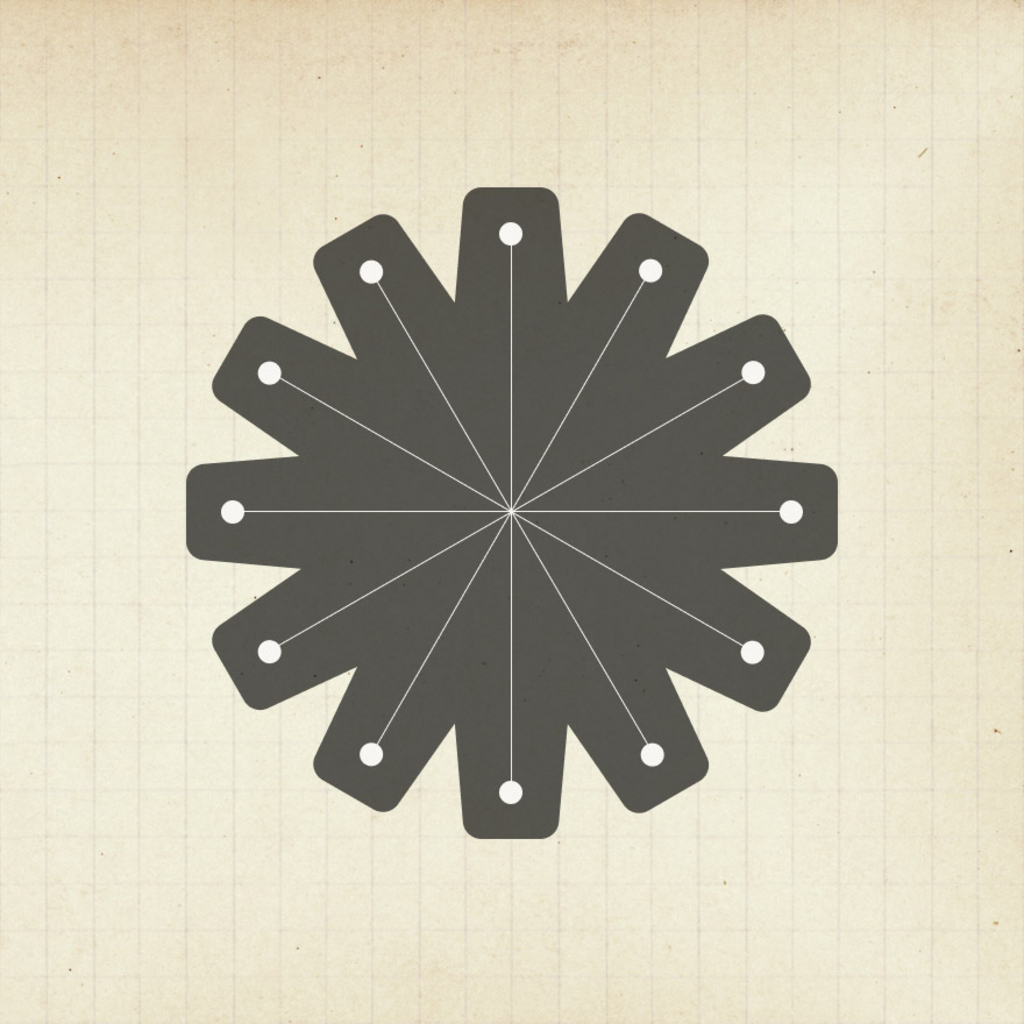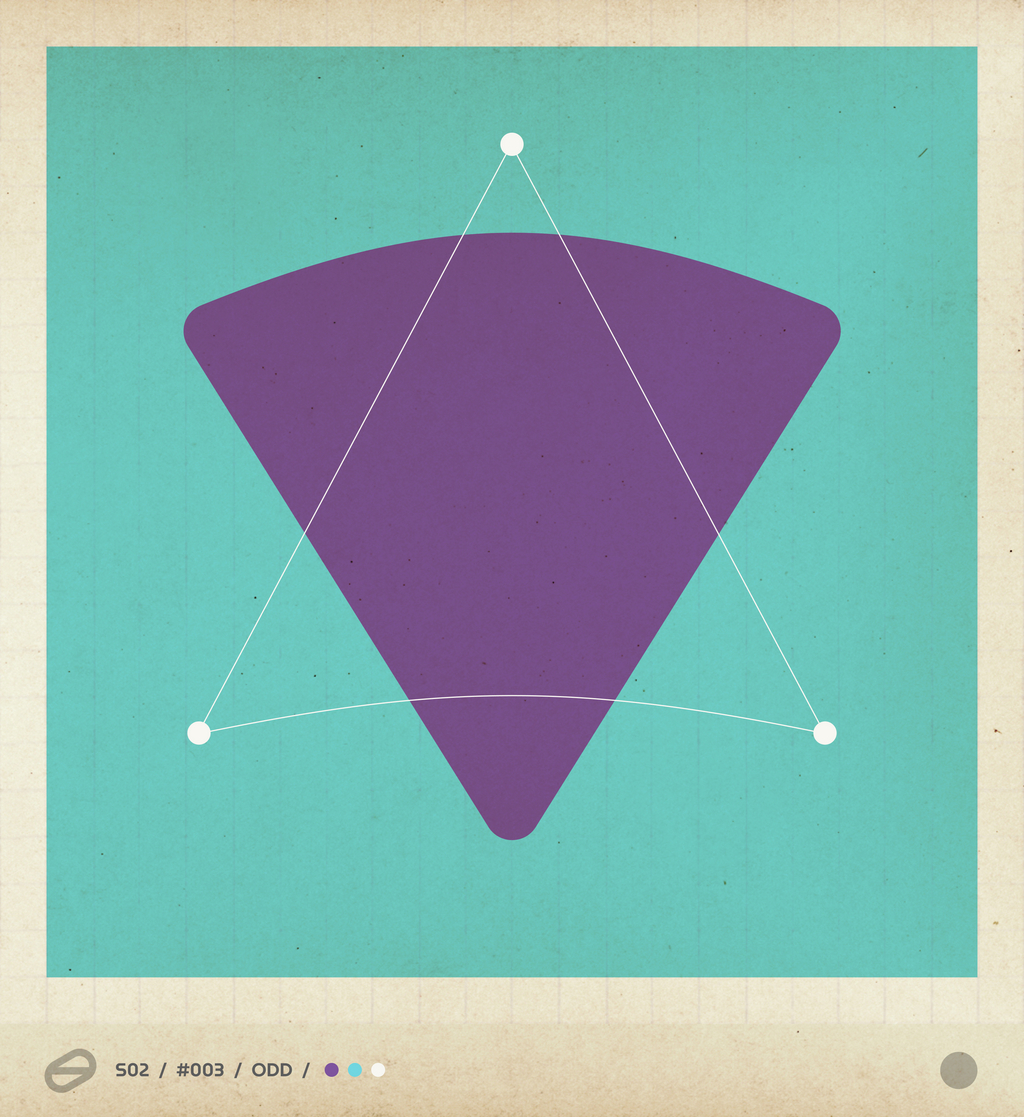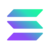This website needs js to run.
Evren\$-

-%Arithmetic - The Number Theory by nanogram One hundred and one handcrafted artwork based on simple mathematics. Each card represents one number as a geometric shape, starting from 1 to 101. Generic numbers are black & white, and all prime numbers are colours. The most common are the even numbers, then the odd numbers; there are only 22 prime numbers (3 colours) and only four magic numbers (4 colours) in this series.234Görünümler

Number Type

Prime

Colours

3

One hundred and one handcrafted artwork based on simple mathematics. Each card represents one number as a geometric shape, starting from 1 to 101. Generic numbers are black & white, and all prime numbers are colours. The most common are the even numbers, then the odd numbers; there are only 22 prime numbers and only four magic numbers in this series.
art
drawing
Arithmetic / 003Lisans: Pub/NonCommMintlenmişSolSea'de6.96
Satın almadan önce her şeyi iki kez kontrol edin!Sahteler nasıl tespit edilir?
• ###### Teklifler
İkincil satışlarda içerik üreticisi telif hakkı ücretleri: 7 %
İlan eden:9hy8...do2B